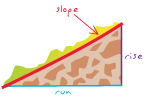# GIS DictionaryBrowse dictionary

## slope

URL copied Share URL
1. [Euclidean geometry] The incline, or steepness, of a surface, measured in degrees from horizontal (0-90), or percent slope (the rise divided by the run, multiplied by 100). A slope of 45 degrees equals 100 percent slope; as a slope angle approaches vertical (90 degrees), the percent slope approaches infinity. The slope of a triangulated irregular network (TIN) face is the steepest downhill slope of a plane defined by the face; the slope for a cell in a raster is the steepest slope of a plane defined by the cell and its eight surrounding neighbors.